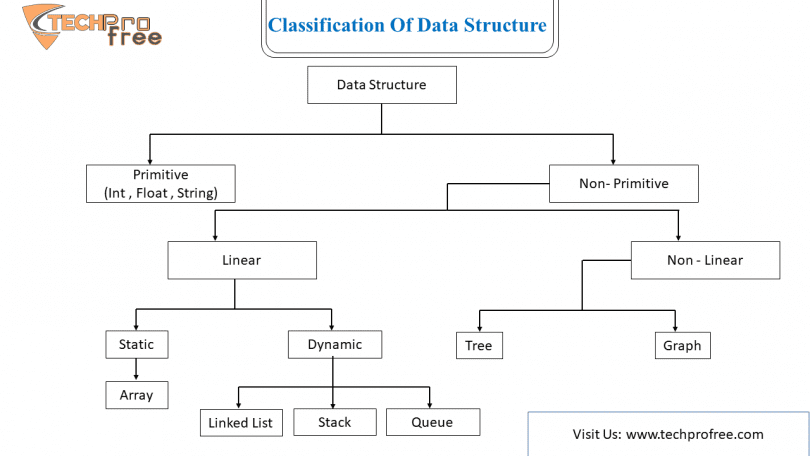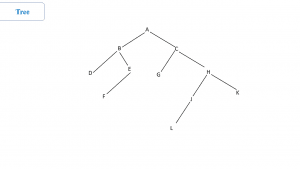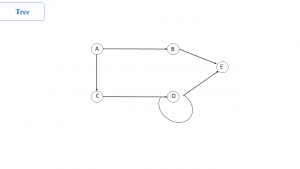# Data Structure From Scratch handwriting Notes For beginnersWhat is Data Structure ? Data can be arranging in many ways, logical and mathematical arrangement of a data is called data structure.

Example

• Array
• Stack
• Queue
• Tree
• Graph and many more

What is Algorithm?

Sequence of steps performed on the data using efficient data structure to solve a given problem.

Example:

• Sorting an array.

Type of Data Structure

1. Primitive and Non-Primitive Data Structure
2. Static and Dynamic Data Structure
3. Persistent and Ephemeral Data Structure

Non-Primitive further divided into two types:

1. Linear Data Structure
2. Non Linear Data Structure

Persistent Further divided into three types:

1. Partially Persistent
2. Fully Persistent
3. Confluently Persistent

Data Structure Operations

The Following four operation playing a major role:

Traversing:

Accessing each records exactly once so that certain items in the records may be processed.

Searching

Finding the location of the record with a given key value.

Inserting:

Adding a new record to a structure.

Deleting

Removing a record to a structure.

Merging:

Combining the record in two different sorted file into a single sorted file.

Sorting:

Arranging the record in some logical order

Searching Algorithm:

A search algorithm is a step by step procedure using to locate specific data among collection of data.

Types of search algorithms with the complexity

Linear Search:

A linea search or sequential search is a method for finding an element within a list.

It is sequentially each element of the list until a match id found or the whole list has been searched.

C(n)=n/2      Complexity of linear search

Binary Search:

In binary search approach the element is always searched in the middle of the portion of an array. Binary search can be implemented only on a stored list items.

If the elements are not stored already we need to store them first.

C(n)=log2n    Complexity of binary search

Arrays:

Arrays is the type of liner data structure. OR

Array is a collection of more than one data but all the data items are same data types, Stord that data in a computer in a continuous memory location.

Memory:

Memory is a log tap of Bytes.

Type of Arrays

One Dimensional Array:

The array with only subscripts that array is called as One Dimensional Array.

Example:

Int a ;      Subscript

Two Dimensional Array:

The array with two subscript that array is called as Two Dimensional Array.

Example:

Int a ;    Subscript

Multi-Dimensional Array:

The array with more than two subscript that array is called as Multi-Dimensional Array.

Link list is a linear data structure. It is also a collection of more than one data items of a dissimilar data type like array but it can note store it in continuous memory location. It can be stored randomly in main memory.

So that link list contains two part one for data and second part for the addresses  of the next data element.

Stacks:

A stack is a list of elements in which an element may be interested or deleted only at one end called the top of the stack.

Push                     Insert element into stack

Delete elements into stack                 Pop

Queue:

A queue is a linear list of elements in which deletions can take place only at one end called front and insertion can place only at the other end called the rear.

Trees

Trees are non-linear data structure where data are stored or data containing a hierarchical relationship between elements.

A binary tree + is defined as finite set of elements.Preorder Inorder Posrorder Ø  Process the root R Ø  Traverse the left tree of R in Preorder. Ø  Traverse the right subtree in Preorder Ø  Traverse left subtree Ø  Process the root R Ø  Traverse right subtree Ø  Traverse left subtree Ø  Traverse right subtree Ø  Process the root R

Graph:

Graph is a collection of two set V and E where,

V                    vertices

E                    edges

Graph is a mathematical structure that represent pair wise relationship between objects where nodes are connected with edges.

Vertex:

Vertex is nothing but data element which is also known as nodes

Edge:

Edge is connection link between two vertices.Representation of Graph: